# Prediction of Wine type using Deep Learning

We use deep learning for the large data sets but to understand the concept of deep learning, we use the small data set of wine quality. You can find the wine quality data set from the UCI Machine Learning Repository which is available for free. The aim of this article is to get started with the libraries of deep learning such as Keras, etc and to be familiar with the basis of neural network.

Before we start loading in the data, it is really important to know about your data. The data set consist of 12 variables that are included in the data. Few of them are as follows –

1. Fixed acidity : The total acidity is divided into two groups: the volatile acids and the nonvolatile or fixed acids.The value of this variable is represented by in gm/dm3 in the data sets.
2. Volatile acidity: The volatile acidity is a process of wine turning into vinegar. In this data sets, the volatile acidity is expressed in gm/dm3.
3. Citric acid : Citric acid is one of the fixed acids in wines. It’s expressed in g/dm3 in the data sets.
4. Residual Sugar : Residual Sugar is the sugar remaining after fermentation stops, or is stopped. It’s expressed in g/dm3 in the data set.
5. Chlorides : It can be a important contributor to saltiness in wine. The value of this variable is represented by in gm/dm3 in the data sets.
6. Free sulfur dioxide : It is the part of the sulfur dioxide that is added to a wine. The value of this variable is represented by in gm/dm3 in the data sets.
7. Total Sulfur Dioxide : It is the sum of the bound and the free sulfur dioxide.The value of this variable is represented by in gm/dm3 in the data sets.

### Step #1: Know your data.

 `# Import Required Libraries  ` `import` `matplotlib.pyplot as plt ` `import` `pandas as pd ` `import` `numpy as np ` ` `  `# Read in white wine data ` `white ``=` `pd.read_csv(``"http://archive.ics.uci.edu/ml/machine-learning-databases/wine-quality/winequality-white.csv"``, sep ``=``';'``) ` ` `  `# Read in red wine data ` `red ``=` `pd.read_csv(``"http://archive.ics.uci.edu/ml/machine-learning-databases/wine-quality/winequality-red.csv"``, sep ``=``';'``) `

First rows of `red`.

 `# First rows of `red` ` `red.head() `

Output: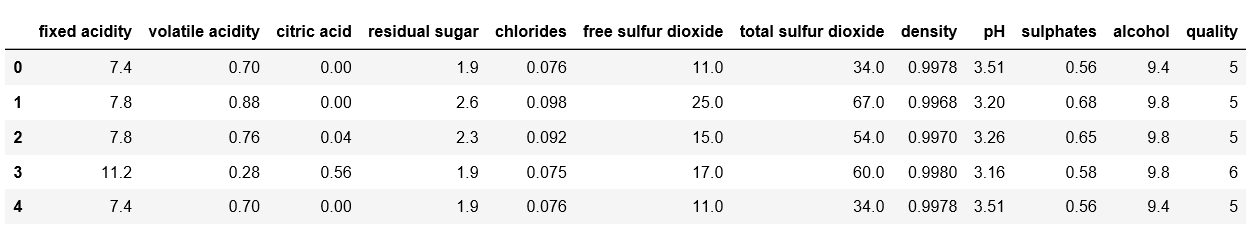Last rows of `white`.

 `# Last rows of `white` ` `white.tail() `

Output: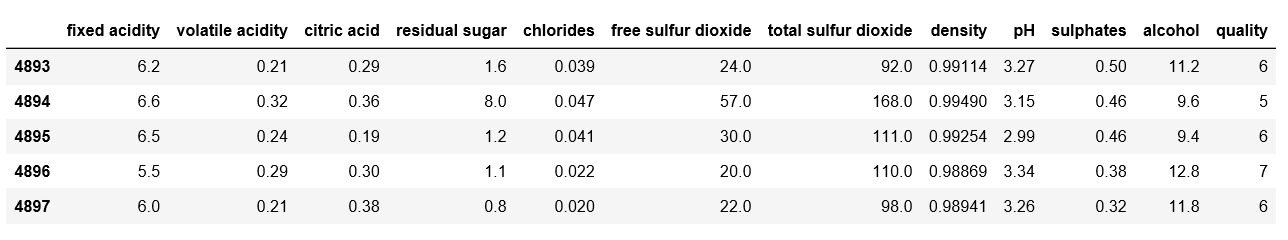Take a sample of five rows of `red`.

 `# Take a sample of five rows of `red` ` `red.sample(``5``) `

Output: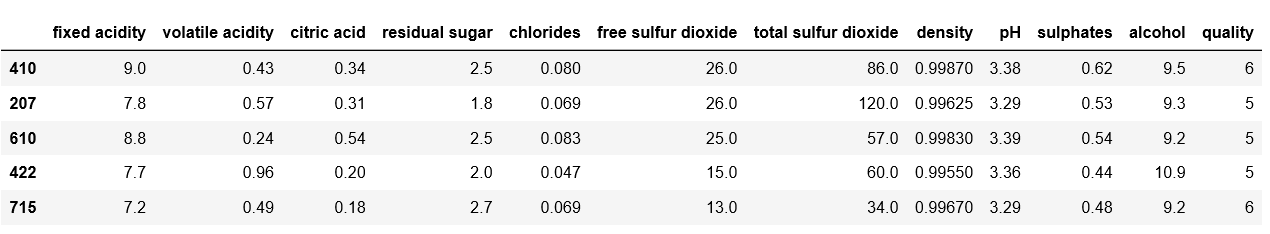Data description –

 `# Describe `white` ` `white.describe() `

Output: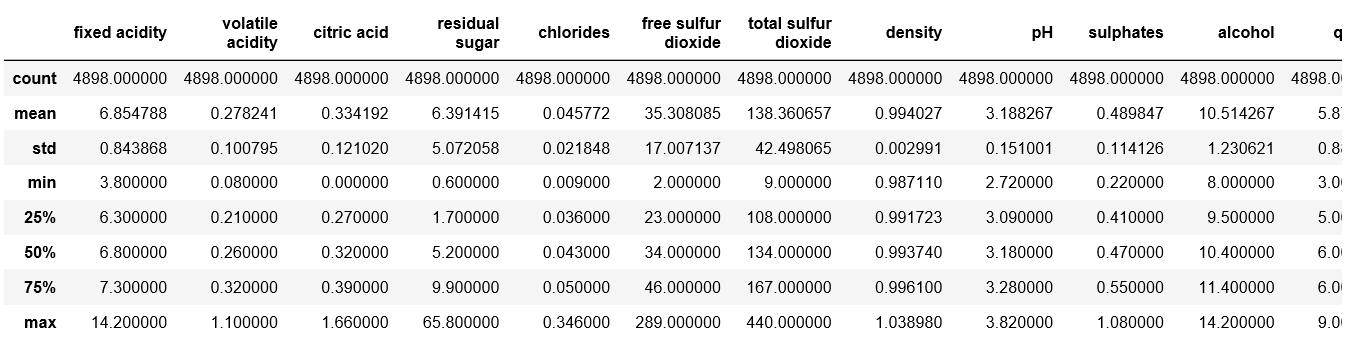Check for null values in `red`.

 `# Double check for null values in `red` ` `pd.isnull(red) `

Output: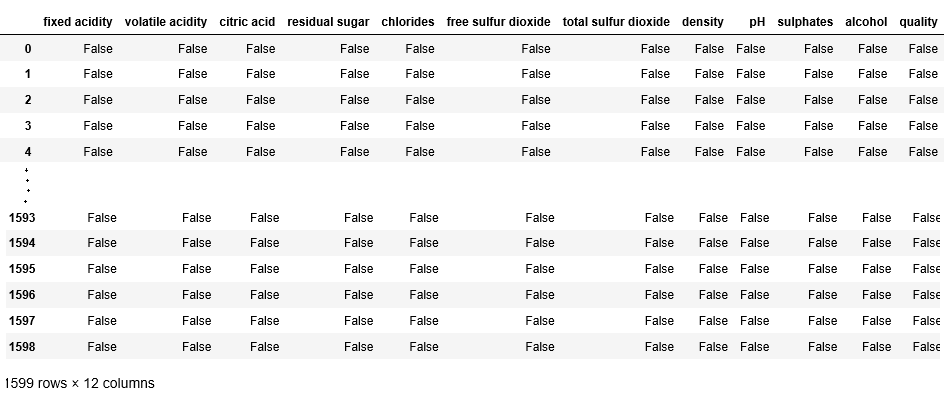### Step #2: Distribution of Alchol.

Creating Histogram.

 `# Create Histogram ` `fig, ax ``=` `plt.subplots(``1``, ``2``) ` ` `  `ax[``0``].hist(red.alcohol, ``10``, facecolor ``=``'red'``, ` `              ``alpha ``=` `0.5``, label ``=``"Red wine"``) ` ` `  `ax[``1``].hist(white.alcohol, ``10``, facecolor ``=``'white'``, ` `           ``ec ``=``"black"``, lw ``=` `0.5``, alpha ``=` `0.5``, ` `           ``label ``=``"White wine"``) ` ` `  `fig.subplots_adjust(left ``=` `0``, right ``=` `1``, bottom ``=` `0``,  ` `               ``top ``=` `0.5``, hspace ``=` `0.05``, wspace ``=` `1``) ` ` `  `ax[``0``].set_ylim([``0``, ``1000``]) ` `ax[``0``].set_xlabel(``"Alcohol in % Vol"``) ` `ax[``0``].set_ylabel(``"Frequency"``) ` `ax[``1``].set_ylim([``0``, ``1000``]) ` `ax[``1``].set_xlabel(``"Alcohol in % Vol"``) ` `ax[``1``].set_ylabel(``"Frequency"``) ` ` `  `fig.suptitle(``"Distribution of Alcohol in % Vol"``) ` `plt.show() `

Output: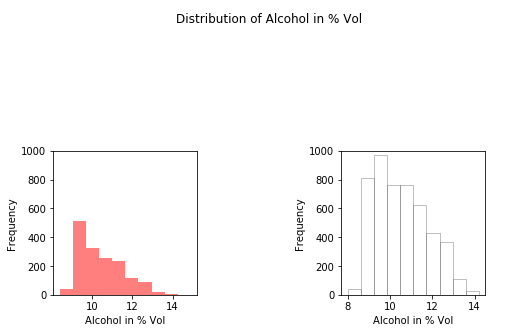Splitting the data set for training and validation.

 `# Add `type` column to `red` with price one ` `red[``'type'``] ``=` `1` ` `  `# Add `type` column to `white` with price zero ` `white[``'type'``] ``=` `0` ` `  `# Append `white` to `red` ` `wines ``=` `red.append(white, ignore_index ``=` `True``) ` ` `  `# Import `train_test_split` from `sklearn.model_selection` ` `from` `sklearn.model_selection ``import` `train_test_split ` `X ``=` `wines.ix[:, ``0``:``11``] ` `y ``=` `np.ravel(wines.``type``) ` ` `  `# Splitting the data set for training and validating  ` `X_train, X_test, y_train, y_test ``=` `train_test_split( ` `           ``X, y, test_size ``=` `0.34``, random_state ``=` `45``) `

### Step #3: Structure of Network

 `# Import `Sequential` from `keras.models` ` `from` `keras.models ``import` `Sequential ` ` `  `# Import `Dense` from `keras.layers` ` `from` `keras.layers ``import` `Dense ` ` `  `# Initialize the constructor ` `model ``=` `Sequential() ` ` `  `# Add an input layer ` `model.add(Dense(``12``, activation ``=``'relu'``, input_shape ``=``(``11``, ))) ` ` `  `# Add one hidden layer ` `model.add(Dense(``9``, activation ``=``'relu'``)) ` ` `  `# Add an output layer ` `model.add(Dense(``1``, activation ``=``'sigmoid'``)) ` ` `  `# Model output shape ` `model.output_shape ` ` `  `# Model summary ` `model.summary() ` ` `  `# Model config ` `model.get_config() ` ` `  `# List all weight tensors ` `model.get_weights() ` `model.``compile``(loss ``=``'binary_crossentropy'``,  ` `  ``optimizer ``=``'adam'``, metrics ``=``[``'accuracy'``]) `

Output: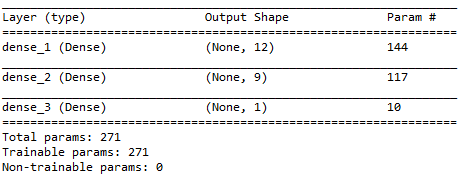### Step #4: Training and Prediction

 `# Training Model ` `model.fit(X_train, y_train, epochs ``=` `3``, ` `           ``batch_size ``=` `1``, verbose ``=` `1``) ` `  `  `# Predicting the Value ` `y_pred ``=` `model.predict(X_test) ` `print``(y_pred) `

Output: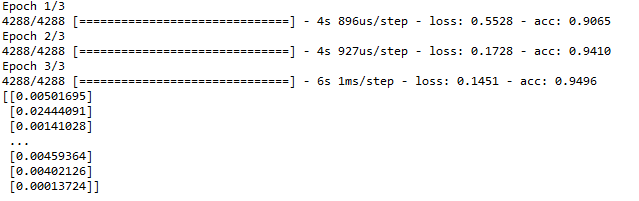My Personal Notes arrow_drop_upCheck out this Author's contributed articles.

If you like GeeksforGeeks and would like to contribute, you can also write an article using contribute.geeksforgeeks.org or mail your article to contribute@geeksforgeeks.org. See your article appearing on the GeeksforGeeks main page and help other Geeks.

Please Improve this article if you find anything incorrect by clicking on the "Improve Article" button below.

Improved By : shubham_singh

Article Tags :

Be the First to upvote.

Please write to us at contribute@geeksforgeeks.org to report any issue with the above content.